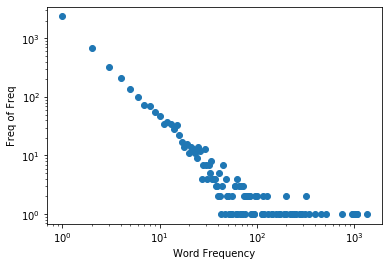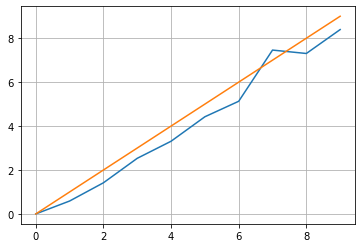# Good_Turing Estimation¶

## Smoothing¶

The occurrence probability of a word can be estimated by the MLE ( maximum likelihood estimator). If a word occurs $r$ times in a corpus, and $N$ is the corpus length. The occurrence probability of the word can be estimated as follows:

$$\hat{P(r)}=\frac{N_r}{N}$$

For a word that does not occur in the corpus, can we say that the occurrence probability of the word is 0? A word does not occur in the training set does not mean that it won't occur in the future. Estimating the probability of unseen words is eseential in NLP. Alan Turing gave a way of estimation: if we scan text sequentially, the probability of encounting a new(unseen) word is

$$\hat{P(0)}=\frac{N_1}{N},$$

Where $N_1$ is the number of words that occur only once (hapex).

## Good_Turing_Smoothing¶

Given a frequency count $r$, the smoothed count $r^*$ is $$r^*=(r+1)\frac{N_{r+1}}{N_{r}}$$

For example,

$${r_1}^*=(1+1)\frac{N_{1+1}}{N_{1}} = 2\frac{692}{2356} =0.58$$
In :
import re
from collections import defaultdict
from collections import Counter
import matplotlib.pyplot as plt

tokens=[]
with open("../data/vldb.txt") as f:
for line in f:
tokens+=(re.findall('[a-zA-Z]+', line.lower()))

N = len(tokens)
C = Counter(tokens)
N_r = Counter(list(C.values()))
print(N_r)

Counter({1: 2356, 2: 692, 3: 327, 4: 207, 5: 137, 6: 101, 7: 74, 8: 69, 9: 56, 10: 47, 12: 37, 11: 35, 13: 34, 15: 33, 14: 28, 16: 22, 17: 17, 19: 16, 21: 14, 18: 14, 25: 14, 29: 13, 22: 13, 26: 12, 23: 11, 20: 11, 24: 9, 34: 8, 28: 7, 30: 7, 32: 7, 45: 7, 41: 5, 33: 5, 35: 4, 48: 4, 37: 4, 63: 4, 31: 4, 36: 4, 27: 4, 65: 3, 60: 3, 68: 3, 39: 3, 59: 3, 44: 3, 72: 3, 38: 3, 95: 2, 323: 2, 51: 2, 49: 2, 127: 2, 200: 2, 50: 2, 80: 2, 101: 2, 56: 2, 117: 2, 83: 2, 74: 2, 78: 2, 85: 2, 40: 2, 79: 2, 42: 2, 77: 2, 1078: 1, 1343: 1, 279: 1, 455: 1, 750: 1, 309: 1, 280: 1, 159: 1, 945: 1, 1010: 1, 1070: 1, 229: 1, 984: 1, 189: 1, 347: 1, 240: 1, 397: 1, 113: 1, 61: 1, 112: 1, 91: 1, 170: 1, 201: 1, 250: 1, 71: 1, 47: 1, 517: 1, 218: 1, 62: 1, 293: 1, 55: 1, 92: 1, 53: 1, 171: 1, 143: 1, 54: 1, 89: 1, 52: 1, 43: 1, 94: 1, 67: 1, 142: 1, 70: 1, 76: 1, 93: 1, 87: 1, 131: 1, 118: 1})

In :
s=sum([k * v for k, v in N_r.items()])
print("Corpus length: "+str(N))
print("voc length: "+str(len(C)))
print("Number of distinct frequency: "+str(len(N_r)))
print("Number of words that occur once: "+str(N_r))
print("Number of words that occur twice: "+str(N_r))
keys=list(N_r.keys()).sort()

Corpus length: 36091
voc length: 4583
Number of distinct frequency: 116
Number of words that occur once: 2356
Number of words that occur twice: 692

In :
plt.loglog(list(N_r.keys()),list(N_r.values()),marker="o", linestyle='None')
plt.xlabel("Word Frequency")
plt.ylabel("Freq of Freq")
plt.show()In :
P_GT=*(len(N_r)+1)
r=*(len(N_r)+1)

r=N_r/N
r=2*N_r/N_r
r=3*N_r/N_r
r=4*N_r/N_r

P_GT=N_r/N
P_GT=2*N_r/N_r/N

print(r)

[0.06527943254550995, 0.5874363327674024, 1.4176300578034682, 2.532110091743119, 0, 0, 0, 0, 0, 0, 0, 0, 0, 0, 0, 0, 0, 0, 0, 0, 0, 0, 0, 0, 0, 0, 0, 0, 0, 0, 0, 0, 0, 0, 0, 0, 0, 0, 0, 0, 0, 0, 0, 0, 0, 0, 0, 0, 0, 0, 0, 0, 0, 0, 0, 0, 0, 0, 0, 0, 0, 0, 0, 0, 0, 0, 0, 0, 0, 0, 0, 0, 0, 0, 0, 0, 0, 0, 0, 0, 0, 0, 0, 0, 0, 0, 0, 0, 0, 0, 0, 0, 0, 0, 0, 0, 0, 0, 0, 0, 0, 0, 0, 0, 0, 0, 0, 0, 0, 0, 0, 0, 0, 0, 0, 0, 0]

In :
import numpy as np
for i in range(len(N_r)):
r[i]=(i+1)*N_r[i+1]/N_r[i] if (N_r[i]>0) else 0
k=10
plt.plot(r[:k])
plt.plot(range(k))
plt.grid()
plt.show()
print("1==>"+str(r))
print("2==>"+str(r))1==>0.5874363327674024
2==>1.4176300578034682

In :



# Project_topics¶

1. Can we apply the Good_Turing smoothing in Naive Bayes classification? Whether GT will improve the performaance?
2. Can we use GT to improve word embedding algorithms?
3. Use GT smoothing for bigram estimation and co-occurrence estimation.

## Resources¶

1. A tutorial on Good-Turing
2. W. A. Gale. Good-Turing Smoothing Without Tears. Journal of Quantitative Linguistics 2: 217-237 (1995).